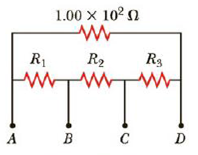Chapter 18, Problem 64AP

Chapter
Section
Textbook Problem

In Figure P18.64, R1 = 0.100 Ω, R2 = 1.00 Ω, and R3 = 10.0 Ω. Find the equivalent resistance of the circuit and the current in each resistor when a 5.00-V power supply is connected between (a) points A and B, (b) points A and C, and (c) points A and D.Figure P18.64

(a)

To determine
The change remained on the 3.0μF capacitor.

Explanation

Given Info:

The battery of the circuit is 12.0V .

The time up to which the circuit is closed is 1.0ms .

The capacitance of the two capacitors are 2.0μF and 3.0μF .

The battery of the circuit is 12.0V .

The time up to which the circuit is closed is 1.0ms .

The capacitance of the two capacitors are 2.0μF and 3.0μF .

The effective capacitance of the circuit is 5.0μF .

The resistance in the circuit is 500.0Ω .

Explanation:

Formula to calculate the effective capacitance is,

Ceff=C1+C2

• C1 is the capacitance of the first capacitor
• C2 is the capacitance of the second capacitor

Substitute 2.0μF for C1 and 3.0μF for C2 to find the effective capacitance,

Ceff=2.0μF+3.0μF=5.0μF

Thus, the effective capacitance of the circuit is 5.0μF .

When the two capacitors are fully charged by the battery and before the circuit is closed, the total charge stored is,

Q0=Ceff(ΔVb) (I)

• ΔVb is the voltage of the battery
• Ceff is the equivalent capacitance

The total stored charge in the capacitors after closing the circuit for 1.0ms is,

q=Q0etτ (II)

• τ is the time constant of the RC circuit
• t is the time that the switch is closed

Since, the time constant of the RC circuit is,

τ=RCeff (III)

• R is the resistance of the circuit

Formula to calculate the total potential difference across the parallel combination of the capacitors is,

ΔV=qCeff (IV)

Re-write the equation (IV) with equations (I), (II) and (III),

ΔV=(Ceff(ΔVb))et(RCeff)Ceff

Substitute 5

(b)

To determine
The charge remained in the 2.0μF capacitor.

(c)

To determine
The current in the resistor in the circuit.

Still sussing out bartleby?

Check out a sample textbook solution.

See a sample solution

The Solution to Your Study Problems

Bartleby provides explanations to thousands of textbook problems written by our experts, many with advanced degrees!

Get Started